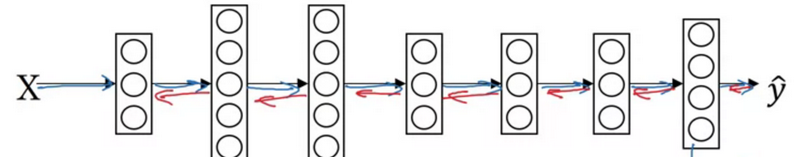# Hyperparameters Tuning and Batch Normalization - Class review

Last updated on：a year ago

Once you have constructed your learning system, you have to tune its hyperparameters. The number of set of parameters means how many models you should train.

# Tuning process

• Hyperparameters: $\alpha, \beta, \varepsilon$.
• Layers
• Hidden units
• Learning rate decay
• Mini-batch size

## Try random value

Don’t use a grid. Picking hyperparameters at random. Grid search can be used for the number of hidden units and layers.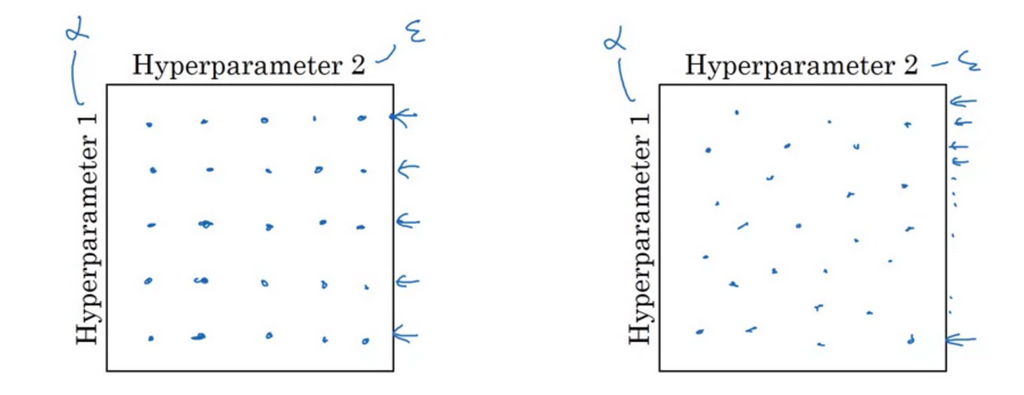Coarse to fine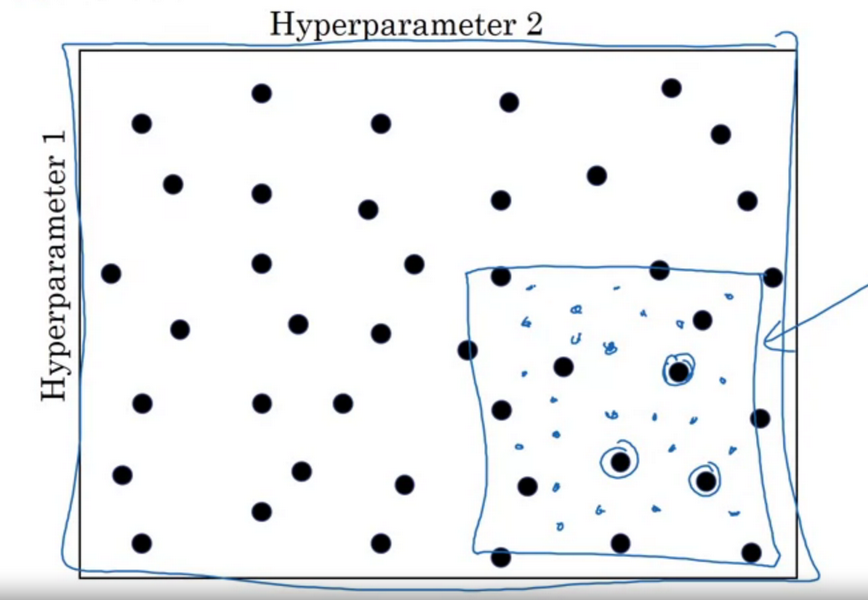## Using an appropriate scale

Appropriate scale for hyperparameters

$$r = -4 * np.random.rand()$$
$$\alpha = np.power(10, r)$$

Exponentially weighted averages, don’t choose it in linear scale

$$choose 1 - \beta$$
$$1 - \beta = 10^r$$
$$\beta = 1 - 10^r$$

It is more sensitive to change $\beta$ when it is close to 1.

$\beta =$ 0.9 0.999
10 1000
$1-\beta =$ 0.1 0.001

$$\beta: (0.9000 \to 0.9005) ~10$$

$$\beta: (0.999) ~ 1000 \to (0.9995) ~ 2000$$

Hyperparameters tuning in practice: Pandas vs. Caviar

Intuition does get scale, re-evaluate occasionally.

• Babysitting one model, Panda
• Training many models in parallel, Caviar

## Normalizing activations in a network

Normalizing inputs to speed up learning, batch norm
$$z^{(i)}_{norm} = \frac{z^{(i)} - \mu}{\sigma^2 + \varepsilon}$$

$$\tilde{z}^{(i)} = \gamma z^{(i)}_{\text{norm}} + \beta$$

If $\gamma = \sqrt(\sigma^2 + \varepsilon)$
$\beta = \mu$

then, $\tilde{z}^{(i)} =z^{(i)}$

Why does batch norm work?

• Learning on shifting input distribution

• Batch norm: no matter how it changes, it eliminates the amount of updating parameters in the earlier layer that can affect the distribution of values.

• The earlier layer doesn’t change much, has the same mean and variance

• Reduce the problem of input value changing, this value becomes more stable

Batch norm at test time
$\mu, \sigma^2$: estimate using exponentially weighted average (across mini-batches)

$$X^1, X^2, X^3, …$$

$$\mu^{1}[l]$$

Using data from training set.

## Fitting batch norm into a neural network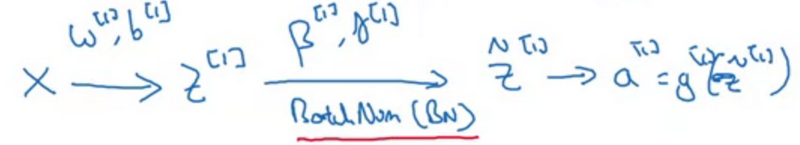$$\beta^{[l]} = \beta^{[l]} - \alpha d\beta^{[l]}$$

Working with mini-batches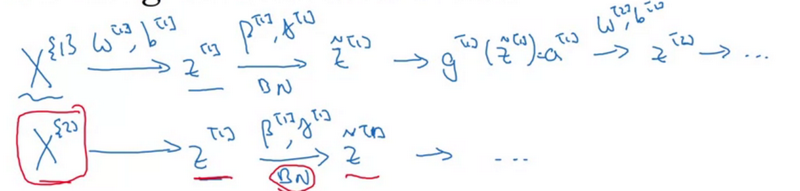Mean subtraction will get rid of the constant.

Parameters:

$$\omega ^{[l]}, \cancel{b^{[l]}}, \beta ^{[l]}, \gamma ^{[l]}$$

$$z ^{[l]} = \omega ^{[l]} a^{[l - 1]} + \cancel{b^{[l]}}$$

## Softmax

Multi-class classification
Softmax layer

$$Z^{[L]} = W^{[L]} a^{[L-1]} + b^{[L]}$$

Activation function

$$t = exp(z^{[L]})$$

$$a^{[L]} = \frac {exp(z^{[L]})} {\sum^4_{i=1} t_i}$$

Training a softmax classifier
Hardmax $[1 0 0 0]^T$
Softmax, more gentle mapping
Softmax regression generalizes logistic regression to C classes.
Loss function
$$L(\hat{y}, y) = - \sum^4_{j=1} y_j \log \hat{y}_j$$
Cost function

$$J(\omega^{}, b^{}, …) = \frac{1}{m} \sum^m_{i=1} L(\hat{y}, y)$$

$$Y = [y^{(1)}, y^{(2)}, …, y^{(l)}]$$
$$\hat{Y} = [\hat{y}^{(1)}, \hat{y}^{(2)}, …, \hat{y}^{(l)}]$$